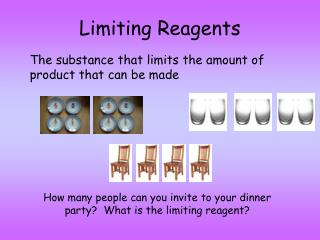DownloadDownload PresentationLimiting Reagents

# Limiting Reagents

Download Presentation## Limiting Reagents

- - - - - - - - - - - - - - - - - - - - - - - - - - - E N D - - - - - - - - - - - - - - - - - - - - - - - - - - -
##### Presentation Transcript

1. Limiting Reagents The substance that limits the amount of product that can be made How many people can you invite to your dinner party? What is the limiting reagent?

2. Sample Problem 2AgNO3 + Cu Cu(NO3)2 + 2Ag What is the limiting reactant when 7.0 g copper react with 25.0 g of silver nitrate? Knowns: Mass of Cu = 7.0 g Mass of AgNO3 = 25.0 g 2 mol AgNO3 = 1 mol Cu Unknowns: Limiting Reagent

3. you HAVE = 0.147 mol AgNO3 1 mol AgNO3 25.0 g AgNO3 ? mol AgNO3 = 170 g AgNO3 1. Find the number of moles of each reactant you HAVE g AgNO3 = ? mol AgNO3 g Cu = ? mol Cu you HAVE = 0.11 mol Cu 1 mol Cu 7.0 g Cu ? mol Cu= 63.5 g Cu

4. 2. Take moles of the 1st reactant and determine the number of moles of the second reactant you NEED. (Use mole ratio from balanced equation.) mol AgNO3 = ? mol Cu 2AgNO3 + Cu Cu(NO3)2 + 2Ag you NEED = 0.074 mol Cu 1 mol Cu 0.147 mol AgNO3 2 mol AgNO3

5. Do you have enough moles of Cu? YES! Just to check, do you have enough moles of AgNO3? NO! you NEED = 0.22 mol AgNO3 0.11 mol Cu 2 mol AgNO3 1 mol Cu So, what is the limiting reagent? AgNO3 (you NEED 0.22 mol and you only HAVE 0.147 mol)

6. Sample Problem What is the limiting reactant when 8.00g HCl reacts with 5.0g Zinc. 2HCl + Zn H2 + ZnCl2 Knowns: Mass of HCl = 6.00 g Mass of Zn = 5.0 g 2 mol HCl = 1 mol Zn Unknowns: Limiting Reagent

7. 1. Find the number of moles of each reactant you HAVE g HCl = ? mol HCl g Zn = ? mol Zn you HAVE = 0.22 mol HCl 1 mol HCl 8.00 g HCl ? mol HCl= 36 g HCl you HAVE = 0.077 mol Zn 1 mol Zn 5.0 g Zn ? mol Zn= 65 g Zn

8. 2. Take moles of the 1st reactant and determine the number of moles of the second reactant you NEED. (Use mole ratio from balanced equation.) mol HCl = ? mol Zn 2HCl + Zn H2 + ZnCl2 you NEED = 0.11 mol Zn 1 mol Zn 0.22 mol HCl 2 mol HCl

9. Do you have enough moles of Zn? NO! So, Zinc is the limiting reagent So…how much ZnCl2 will you form?

10. Percent Yield When 84.8g of iron (III) oxide reacts with an excess of carbon monoxide, 54.3g of iron is produced. Fe2O3 + 3CO 2Fe + 3CO2 1. Find theoretical yield (change grams of reactant that you’re starting with to grams of the product that you want to find) 84.8 g Fe2O3 1 mol Fe2O3 2 mol Fe 56 g Fe = 59.4 g Fe 160 g Fe2O3 1 mol Fe2O3 1 mol Fe

11. % Yield = (actual / theoretical) * 100% 54.3 g Fe = 91.4% Fe recovered % Yield = * 100% 59.4 g Fe